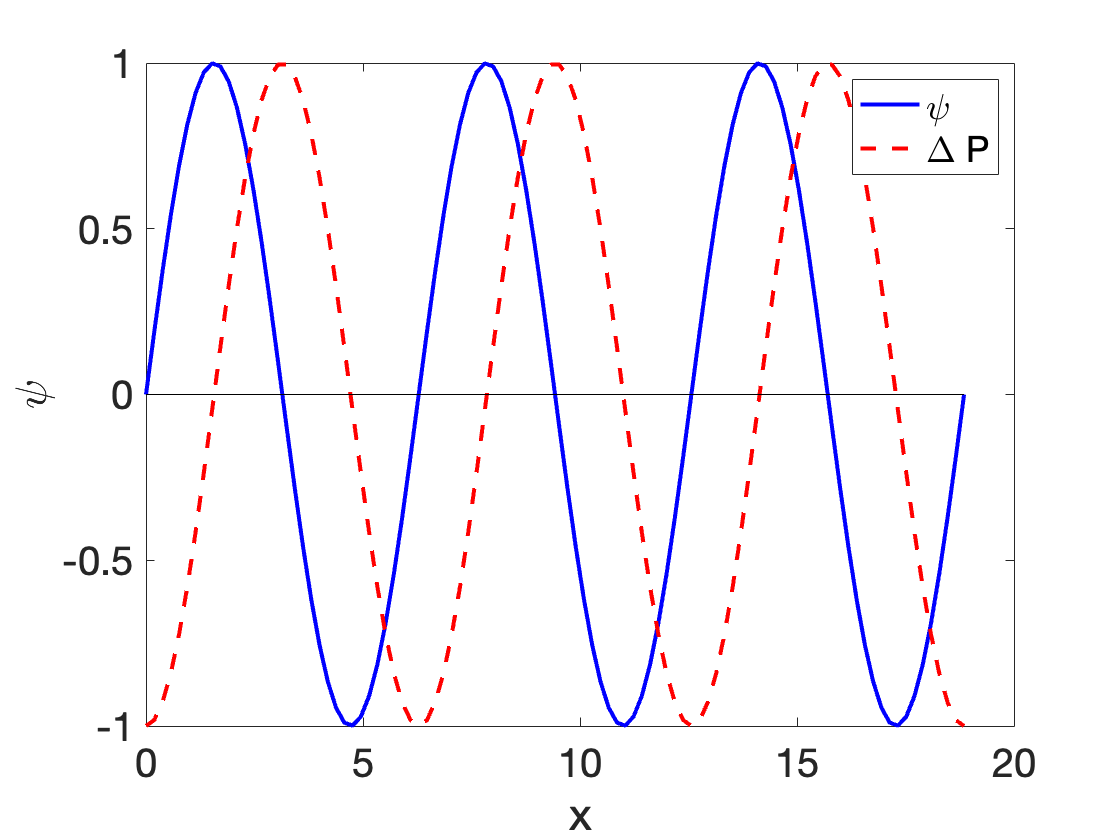## Section15.2Planar Sound Wave

Consider a planar sound wave in air of frequency $f$ and wavelength $\lambda\text{.}$ We can think of this wave in terms of displacement of particles of the air or in terms of presser differences at different places. Let us first look at the displacement wave.

Let direction of wave be towards the positive $x$ axis and amplitude of the wave at the origin be $0$ at $x=0$ and $t=0\text{.}$ Then displacement at $x$ at intant $t$ will be give by

\begin{equation} \psi(x,t) = D_0 \sin\left( \frac{2\pi}{\lambda} x - 2\pi f t\right),\label{eq-sound-displacment-plane-wave}\tag{15.2.1} \end{equation}

where $D_0$ is the amplitude of the wave. We use letter $D$ for the amplitude instead of $A$ because we will use $A$ for area below. The wavenumber $k$ and angular frequency $\omega$ of the wave are

\begin{equation*} k = \frac{2\pi}{\lambda},\ \ \omega = 2\pi f. \end{equation*}

To obtain the corresponding pressure wave, we need to look at a small volume through which this displacment wave is passing and apply the definition of Bulk modulus $B$ from the chapter on Statics. The pressure change $\Delta P$ for a fractional volume change $\Delta V / V$ is related by Bulk modulus $B$ by

\begin{equation*} \Delta P = - B\frac{\Delta V}{V}. \end{equation*}

Consider a small box of thickness $\Delta x$ along the wave and area $A$ perpendicular to the wave. Suppose as a result of the displacement wave $\psi\text{,}$ this box is shrunk by $\Delta \psi\text{.}$ this shrinking will happen due to different values of $\psi$ at the two ends of the box separated by $\Delta x\text{.}$Thus, volume change will be $\Delta V = A\Delta \psi$ while the original volume was $A\Delta x\text{.}$ From the definition of bulk modulus in chapter on statics, you will note that the pressure in the box will change by $\Delta P$ given by

\begin{equation*} \Delta P = - B\frac{\Delta \psi}{\Delta x}. \end{equation*}

In the limit of $\Delta x \rightarrow 0$ this becomes a partial derivative with respect to $x\text{.}$

\begin{equation} \Delta P = - B\frac{\partial \psi}{\partial x}.\tag{15.2.2} \end{equation}

Using $\psi$ from Eq. (15.2.1) we get

\begin{equation} \Delta P = - \frac{2\pi}{\lambda} D_0 B \cos\left( \frac{2\pi}{\lambda} x - 2\pi f t\right).\tag{15.2.3} \end{equation}

We can write this as a sine function to compare with the displacement wave as

\begin{equation} \Delta P = P_0 \sin\left( \frac{2\pi}{\lambda} x - 2\pi f t - \frac{\pi}{2}\right),\tag{15.2.4} \end{equation}

where $P_0$ is the amplitude of the pressure wave

\begin{equation*} P_0 = \frac{2\pi}{\lambda} D_0 B. \end{equation*}

Note that the argument of sine for pressure wave has a phase constant of $-\pi/2$ as compared to the displacement wave give in Eq. (15.2.1). Thus, pressure wave has peaks and troughs when displacement wave is zero and vice versa as shown in Figure 15.2.1.Figure 15.2.1. Comparison of displacement wave $\psi$ (the solid line) and pressure wave $\Delta P$ (the dashed line) in space at one instant.